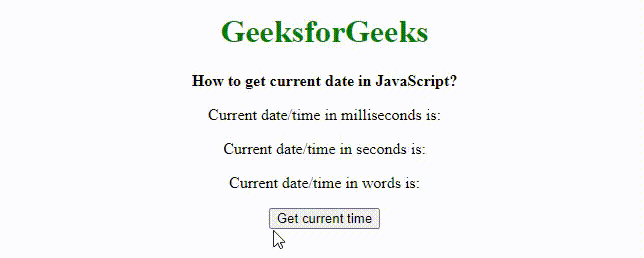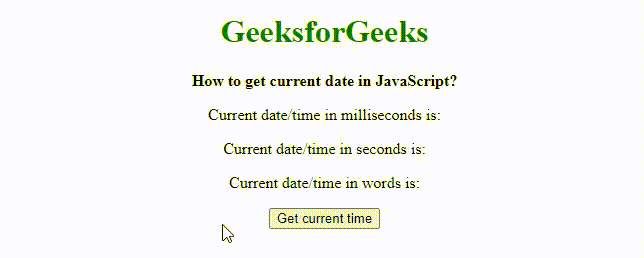Open in App
Not now

# How to get the current date and time in seconds ?

• Last Updated : 11 Nov, 2021

In this article, we will learn how to get the current date & time in seconds using Javascript build-in methods. We will perform it in 2 ways:

• Using Date.now() method
• Using new Date.getTime() method

Method 1: Using Date.now() method

The Date.now() method returns the number of milliseconds elapsed since January 1, 1970, 00:00:00 UTC. This is known as the epoch time. It may be used for timestamping as an order of events could easily be checked by comparing the timestamps. The returned milliseconds can be converted to seconds by dividing the value by 1000 and then using the Math.round() function to round the value. This is done to prevent inconsistencies due to the float values.

Syntax:

`Date.now();`

Parameters: This method does not accept any parameter.

Return Values: It returns the number of milliseconds elapsed since January 1, 1970, 00:00:00 UTC.

Example: This example describes getting the current date & time using Date.now() method.

## HTML

 ```<``html``>`` ` `<``head``>``    ``<``title``>How to get current date in JavaScript?```` ` `<``body` `style``=``" text-align:center;"``>``    ``<``h1` `style``=``"color: green;"``>``        ``GeeksforGeeks``    `` ``    ``<``b``>``        ``How to get current date in JavaScript?``    ````    ``<``p``>``        ``Current date/time in milliseconds is: ``        ``<``span` `class``=``"output-msecs"``> ``    ```` ` `    ``<``p``>``        ``Current date/time in seconds is: ``        ``<``span` `class``=``"output-secs"``> ``    ```` ` `    ``<``p``>``        ``Current date/time in words is: ``        ``<``span` `class``=``"output-words"``> ``    ```` ` `    ``<``button` `onclick``=``"getCurrentDate()"``>Get current time``     ` `    ``<``script` `type``=``"text/javascript"``>``    ``function getCurrentDate() {``        ``dateInMillisecs = Date.now();``         ` `        ``// rounding the value to prevent inconsistencies``        ``// due to floating points``        ``dateInSecs = Math.round(dateInMillisecs / 1000);``        ``dateInWords = new Date(dateInMillisecs);``        ``document.querySelector('.output-msecs').textContent = ``        ``dateInMillisecs;``        ``document.querySelector('.output-secs').textContent = ``        ``dateInSecs;``        ``document.querySelector('.output-words').textContent = ``        ``dateInWords;``    ``}``    `````` ` ``

Output:Date.now() Method

Method 2: Using new Date.getTime() method

The Date.getTime() method returns the number of milliseconds elapsed since January 1, 1970, 00:00:00 UTC. It always uses the UTC for time representation. It has to be initialized with the new keyword, unlike the Date.now() method. The returned milliseconds can be converted to seconds by dividing the value by 1000 and then using the Math.round() function to round the value. This is done to prevent inconsistencies due to the float values.

Syntax:

`new Date().getTime();`

Parameters: This method does not accept any parameter.

Return type: A numeric value equal to no of milliseconds since Unix Epoch.

Example: This example describes getting the current date & time using Date.getTime() method.

## HTML

 ```<``html``>`` ` `<``head``>``    ``<``title``>How to get current date in JavaScript?```` ` `<``body` `style``=``" text-align:center;"``>``    ``<``h1` `style``=``"color: green"``>``        ``GeeksforGeeks``    ````    ``<``b``>``        ``How to get current date in JavaScript?``    ````    ``<``p``>``        ``Current date/time in milliseconds is: ``        ``<``span` `class``=``"output-msecs"``> ``    ```` ` `    ``<``p``>``        ``Current date/time in seconds is: ``        ``<``span` `class``=``"output-secs"``> ``    ```` ` `    ``<``p``>``        ``Current date/time in words is: ``        ``<``span` `class``=``"output-words"``> ``    ```` ` `    ``<``button` `onclick``=``"getCurrentDate()"``>Get current time``     ` `    ``<``script` `type``=``"text/javascript"``>``    ``function getCurrentDate() {``        ``dateInMillisecs = new Date().getTime();``       ` `        ``// Rounding the value to prevent inconsistencies``        ``// due to floating points``        ``dateInSecs = Math.round(dateInMillisecs / 1000);``        ``dateInWords = new Date(dateInMillisecs);``        ``document.querySelector('.output-msecs').textContent = ``        ``dateInMillisecs;``        ``document.querySelector('.output-secs').textContent = ``        ``dateInSecs;``        ``document.querySelector('.output-words').textContent = ``        ``dateInWords;``    ``}``    `````` ` ``

Output:Date.getTime() Method

My Personal Notes arrow_drop_up